## General Chemistry

The oxidation state (or oxidation number) is assigned to each atom in a compound as an indicator of whether the atom is neutral, electron-rich, or electron-poor.

The general principle is that the more electronegative atom pulls the electron density of the bond toward it and thus it is electron-rich while the other atom is electron-poor.

For example, in water the oxygen is connected to two hydrogen atoms. Remember, oxygen is more electronegative than hydrogen, and therefore, it is electron-reach while the hydrogens are electron-poor. Each bond with a hydrogen brings the oxygen -1 oxidation state, so two O-H bonds make its oxidation state -2. Each hydrogen has a +1 oxidation state.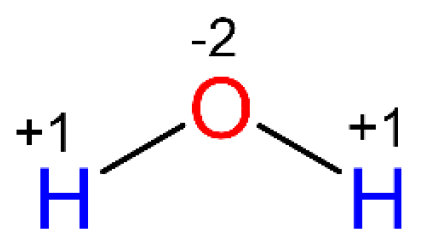Oxygen has a higher affinity for electrons, so it pulls one electron from each bond. Therefore, the oxidation number of oxygen is -2.

Hydrogen has a lower affinity for electrons, and it gives the electron to oxygen, thus the oxidation number of hydrogen in +1.

• The oxidation state is not an actual charge, but rather an assigned, descriptive number.

# Rules for Assigning Oxidation Numbers

1) The oxidation state of an atom in a free element is 0.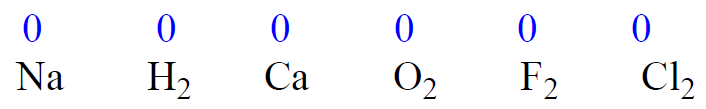2) The oxidation state of a monoatomic ion is equal to its charge.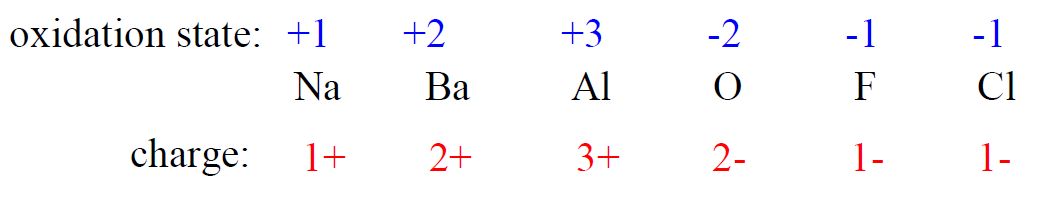3) The sum of the oxidation states in a neutral molecule is 0.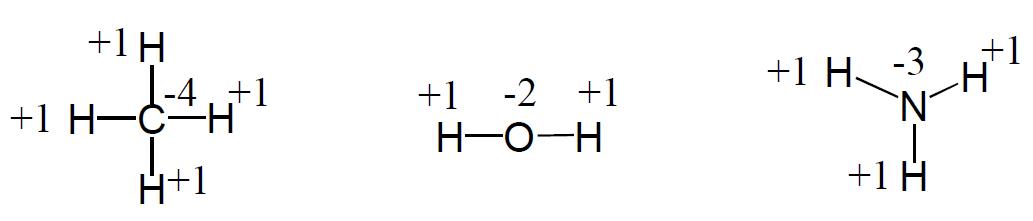4) The sum of the oxidation states in an ion is equal to the charge of the ion.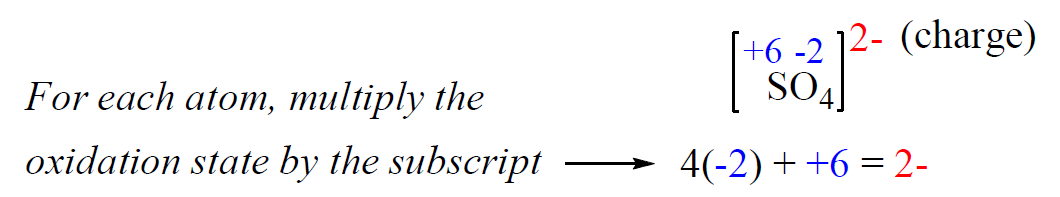5) Metals have positive oxidation states. Group 1A metals: +1, group 2A metals: +2.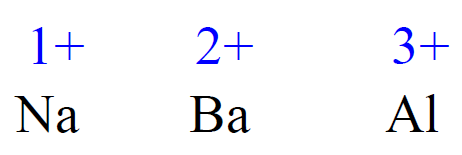6) In compounds with nonmetals, hydrogen is assigned +1 as its oxidation number. See rule 3.

7) In its compounds, fluorine is always assigned -1 as its oxidation number. For example,  HF, CaF2, AlF3

8) In most compounds, the oxidation of oxygen is -2. Example: H2O, CO2, NaOH# Determining Oxidation States

To determine the oxidation number of an atom(s) in a molecule or an ion, start with the known oxidation numbers, and keep in mind that the summary is zero for neutral molecules and is equal to the charge for ions.

For example, what is the oxidation number of the carbon in CO2?

Rule 8 – The oxidation number of oxygen is -2, so put -2 on oxygen and assign x for the oxidation state of C.After, this we set up an equation: the subscript of each atom is multiplied by its oxidation number, and the sum of this products must be equal to zero because CO2 is a neutral molecule:

x + 2(-2) = 0, x = +4

Therefore, the oxidation number of carbon in CO2 is +4. So, one carbon with +4 oxidation number balances the -4 coming from two oxygens, thus making the sum of oxidation states zero.

# Oxidation States in Ions

Determine the oxidation state of sulfur in the SO42- ion.

Rule 8 – The oxidation number of oxygen is -2, so put -2 on oxygen and assign x for the oxidation state of S.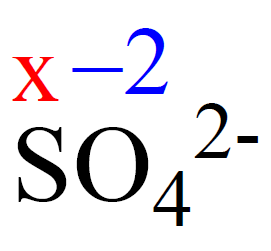After, this we set up an equation: the subscript of each atom is multiplied by its oxidation number, and the sum of this products must be equal to the charge of the ion (-2 or 2-) because SO42- is an ion:

x + 4(-2) = -2, x = +6

Therefore, the oxidation number of sulfur is +6.

Check Also

#### Practice

1.

Determine the oxidation state of sulfur and oxygen in SO2

S +4
O -2

2.

Determine the oxidation state of sulfur and oxygen in sulfate (SO42-) ion

S +6
O -2

3.

Determine the oxidation state for each element in Mg3(PO4)2

Mg +2
P +5
O -2

4.

Determine the oxidation state for each element in Na2Cr2O7